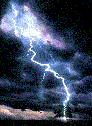### Physics 1B Thermal Physics & Electromagnetism

`H. E. Smith                                                                                                       Spring 2000`Physics 1B - Reading Quiz #81. When the switch is closed in Circuit A above; what happens in the resistor in Circuit B?
• A. a momentary current flows from left to right.
• B. a constant current flows from left to right as long as the switch is closed.
• C. a momentary current flows from right to left.
• D. a constant current flows from right to left as long as the switch is closed.
• E. nothing.

2. It is desirable to transmit electrical energy at high voltage because
• A. DC power, which is the easiest means of generating power, naturally favors high voltage.
• B. resistive power losses, P=I2R, will be minimized.
• C. power transmitted, P=I2R, will be maximized.
• D. high voltage is desirable for household electrical circuits.
• E. it is important to get power lines as high above the ground as possible, thus high voltage is required.